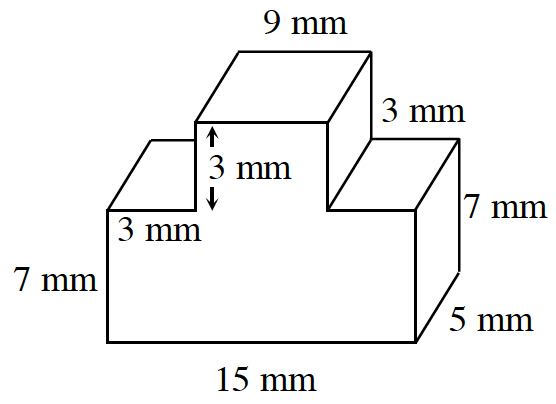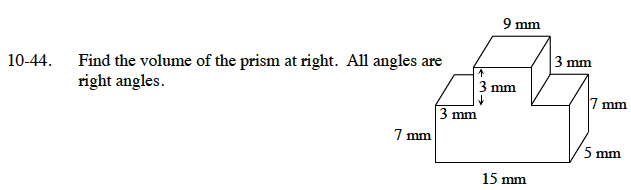### Home > CC3 > Chapter Ch10 > Lesson 10.1.3 > Problem10-44

10-44.
1. Find the volume of the prism below. All angles are right angles. Homework Help ✎

2.Find the area of the base (which is the side facing you) and multiply by the height of 5 mm.

The base can be divided into two rectangles. One that is 15 mm by 7 mm and one that is 9 mm by 3 mm.

Find the area of each smaller rectangle and add them together.

Volume of a prism = (area of base)(height)
Now multiply the area you found for the base by the height to get the total volume of the prism.

660 mm³